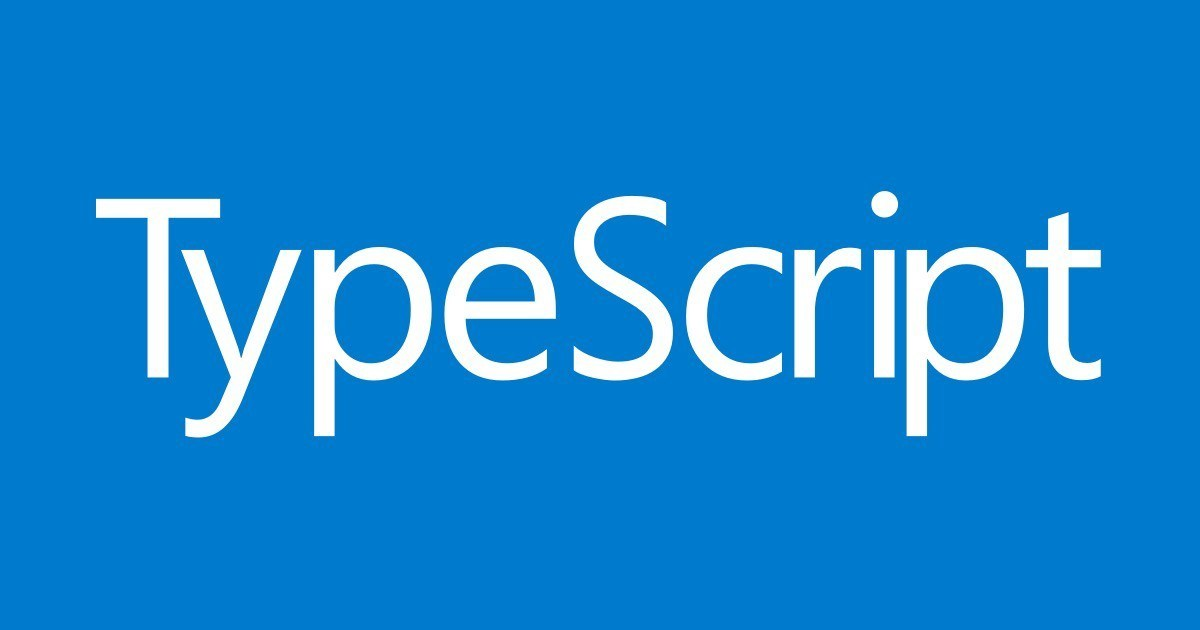## TypeScript学习笔记TypeScript入门命令npm i typescipt -g tsc src_file.ts声明和类型let a:number; a=12; a='12';//报错 let b=123; b='123';//报错 会自动赋值类型 //函数 function sum(a:number,b:number):number{ return a+b; } let c:11;//字面量数据类

- 阅读全文 -

- 阅读全文 -

## for循环我不知道的事情

for循环中，定义的东西，居然是赋值到了所出的作用域内for( var i=0 ; i<1 ; i++ ){ var a=0; } console.log(i) // 1 console.log(a) // 0 知道和理解完全是两回事，知道是我知道ES6之前，只有全局作用域和函数作用域，而我一直没有去理解它现在终于明白了，理解了，知道为什么ES

- 阅读全文 -

- 阅读全文 -

## JS实现深拷贝

//深拷贝的实现方法，不支持函数 let arr=[0,1,2,{a:"a",b:"b",c:"c"}]; let arr2=clone(arr); function clone(obj){ let objType=checkType(obj); let temp; if( objType==="Array" ){ temp=[]; }else if( objType=

- 阅读全文 -